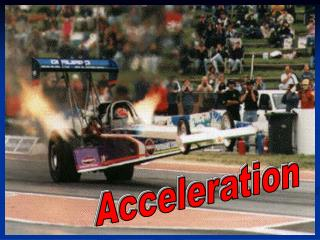DownloadDownload PresentationAcceleration

# Acceleration

Télécharger la présentation## Acceleration

- - - - - - - - - - - - - - - - - - - - - - - - - - - E N D - - - - - - - - - - - - - - - - - - - - - - - - - - -
##### Presentation Transcript

1. Acceleration

2. Change in Velocity • You are probably most familiar with the velocity changes of a moving bus or car. • The rate at which velocity (speed or direction) changes occur is called acceleration.

3. Acceleration= final velocity- starting velocity time Change in velocity = final – starting velocity velocity Acceleration= change in velocity time

4. Problem time! • A car travelling at 60 km/s to the east accelerates to 90 km/s to the east in 3 s. What is the car’s acceleration? • Step 1: What we know! • Starting velocity Vi: 60 km/s, east • Final velocity Vf: 90 km/s, east • Time t: 3 s • Step 2: What equation/what are we finding? • a = (Vf – Vi)/t

5. Problem continued! • Step 3: Put data into equation: • a = (9 km/s – 6 km/s)/3 s • We have chosen east as positive! • Step 4: Do the math! • a= (3 km/s)/3 s • a= 1 km/s2 • Step 5: Check the units and direction!

6. Positive acceleration Negative acceleration

7. Another Problem! • A car travelling at 60 km/s north slams on the brakes to avoid hitting a deer. The car comes to a safe stop 6 s later. What is the car’s acceleration? • Step 1: What we know: • Vi: 60 km/s north • Vf: • t: 6 s

8. Problem 2 Continued! • Step 2: What equation/what are we trying to find? • a = (Vf – Vi)/t • Step 3: Substitute the numbers in the equation: • a = (0 km/s – 60 km/s)/6 s • Step 4: Do the math! • a = (- 60 km/s)/6 s • a = - 1 km/s2 • What does the negative mean? • Step 5: Check the units and direction.

9. Constant acceleration produces a straight line when velocity and time are graphed • The slope of the line tells us the acceleration

10. What if we need to know something else? • A car experiences an acceleration of -3 km/s for 6 s. The car’s initial velocity was 60 km/s to the south. What is its final velocity? • What can we do? • Step 1: What do we know? • Vi = 60 km/s • Vf = ? • a = - 3 km/s • t = 6 s

11. Step 2: What equation/what are we trying to find? • Start with what we know: • a = (Vf – Vi)/t • Rearrange: • at = (Vf – Vi) • So at + Vi = Vf • Check to make sure the equation makes sense! • Vf = Vi + at

12. Step 3: Put the data in! • Vf = 60 km/s + (-3 km/s2)(6 s) • Step 4: Do the math. • Vf = 60 km/s + (-18 km/s) • Vf = 42 km/s, south • Step 5: Check the units (and direction).

13. Acceleration is fun! Gallardo LP560